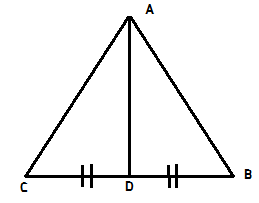# Important theorems related to triangles

The following four theorems are most important in solving questions on triangles.

## Pythagoras’ theorem:

In a right angle triangle the square of the hypotenuse is equal to the sum of the squares of other two sides.${b^2} = {a^2} + {c^2}$
Pythagorean triplet: There are certain triplets which satisfy the pythagoras’ theorem and are common, called pythagorean triplet. For example: 3, 4, 5;    5, 12, 13;    10, 24, 26;    7, 24, 25;    8, 15, 17

## Appolonious theorem:In triangle ABC, AD is median, which divides BC into two equal parts.
$\therefore$ $A{B^2} + A{C^2} = 2(A{D^2} + B{D^2}) = 2(A{D^2} + D{C^2})$

## Stewart theorem:In Triangle ABC, AD divides side BC in the ratio m and n. (Here AD need not be median)
$\therefore m.{b^2} + n.{c^2} = a({d^2} + mn)$

## Mean proportionality and Mid Point theorem:In the first triangle, $DE // BC$ so $\displaystyle\frac{{AD}}{{DB}} = \frac{{AE}}{{EC}}$
In the second triangle, D and E are mid points of  AB and AC respectively.
Which implies, $\displaystyle\frac{{AD}}{{DB}} = \frac{{AE}}{{EC}} = 1$
Also, $DE = \displaystyle\frac{1}{2}BC$